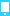# GRE数学算术重点类题目练习

2019-4-29 11:33:00   无忧考网     [手机版 ] [ 投诉建议 ] [ Word文档下载 ]
GRE网发布GRE数学算术重点类题目练习，更多GRE数学算术重点类题目练习相关信息请访问无忧考网GRE留学外语考试频道。

【导语】学而不思则罔，在掌握知识点之后将其运用在解题中才是学习的好方法。备考需要一点点积累才能到达效果，无忧考网为您提供了“GRE数学算术重点类题目练习”，欢迎阅读参考！更多相关讯息请关注无忧考网！比较大小

A. If the quantity in column A is greater;

B. If the quantity in column B is greater;

C. If the two quantities are equal;

D. if the relationship cannot be determined from the information given.

题目：

1.How many positive whole numbers less than 81 are not equal squares of
whole numbers?

A.9

B.70

C.71

D.72

E.73

答案：D

2. 23(784)

24(783)

答案：B

3.A printer numbered consecutively the pages of a book, beginning with 1 on
the first page. In numbering the pages, he printed a total of 189 digits.

The number of pages in the book

100

答案：B

4. m,p, and x are positive integers and mp=x.

m

x

答案：D

The number of distinct positive factors of n

10

答案：B

6.Seven is equal to how many thirds of seven?

A.1/3

B.1

C.3

D.7

E.21

答案：C

7.How many positive integers less than 20 are equal to the sum of a
positive multiple of 3 and a positive multiple of 4?

A.2

B.5

C.7

D.10

E.19

答案：D

8.5/2 percent of 1120

22*7

答案：C

9.The figure above consists of 25 squares. If the figure were folded along
the dotted diagonal to forn a flat triangle, then 26 minus the number in the
square that would coincide with the square containing 26 would be

A.13

B.14

C.15

D.16

E.17

答案：D

10.What is the remainder when 63 is divided by 8?

A.5

B.3

C.2

D.1

E.0

答案：E